# Excel VBA Range object, properties and methods

Excel VBA Range object represents a cell or group of cells. For example, you can say A1 as a range as well as A1:C40 also as a range. It is one of the most important object of Excel VBA. Let us see the Range object methods and properties with numerous examples.

### How to assign a value to a cell using Excel VBA range object?

Just assume that you want to enter a value 10 in cell B5 of sheet1 in the workbook. Go to Developer tab-> Visual basic->Insert-> Module. In the Module1, enter the below code and press F5 to execute.

Code

`````` Sub assign()

ThisWorkbook.Sheets(1).Range("B5").Value = "10"

End Sub``````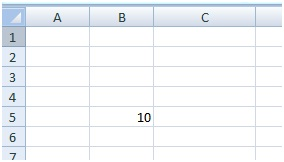Thisworkbook– It refers the workbook where this code resides.

Sheets(1)– It refer the first sheet “Sheet1” . You can locate the sheet either by name or by index. Here, Sheets(1) refer the first sheet by its index value 1. If you want to locate the sheet by its name then you should specify the sheet name inside the double quotes. For e.g. Sheets(“Sheet2”)

Range(“B5”)– It refer the B5 of sheet 1.It must always go inside the double quotes.

Value– It is a property of range object used to set value to the specified range. Here it’s take the value of B5. You can still see value 10 when you remove the “.Value”.

“=” is an assignment operator used to set value on its right to the specified range on its left.

### How to assign same value to more than one cell using Excel VBA range object?

Let us assume an user want to print text “I’m new to VBA” in the Range C3:F7.

Code

``````Sub assign()

ThisWorkbook.Sheets(1).Range("C3:F7").Value = "I'm new to VBA"

End Sub``````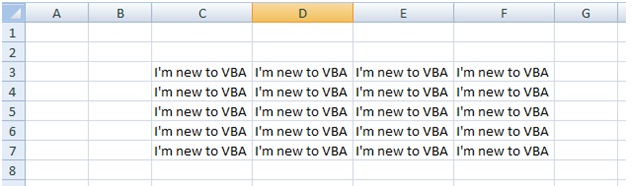Range(“C3:F7”) define the cells starting from C3 to F7 and assign the text “I’m new to VBA”.

### How to assign value to named range using Excel VBA range object?

Let select the range A1:D6. Click the Name box at the top left and name it as myRange as shown in the below fig. Now you set a name to range A1:D6. So instead of representing  as A1:D6 you can call by its name “myRange”

Code

`````` Sub assign()

ThisWorkbook.Sheets(1).Range("myRange").Value = "I'm good with VBA"

End Sub

``````

###How to insert formula to a cell using Excel VBA Range object?

Let’s say that we have numerical values in the Range A1:A9 and we want to add all those values and display the sum in A10 using the Sum function. Using Formula property we can enter formula or functions in VBA.

Code

``````Sub formula()
ThisWorkbook.Sheets(1).Range("A10").formula = "=Sum(A1:A9)"
End Sub

``````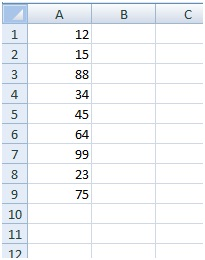Comments

When you double click the cell A10, you could see that VBA code insert Sum function rather than showing the sum value of cells A1:A9 as shown in the below Fig.``````

### How to refer range using cells method?

Range object also has a method called cells. Cells method returns the range object when evaluated. Cells method take two arguments. Cells(x,y) where x represent the row and y represent the column. For e.g. Sheets(1).Cells(3,5) represent the Cell “E3”, Cells(1,2) represent the Cell “B1”. Cells method can also be used to refer group of cells.

Code

``````Sub cells_method()
ThisWorkbook.Sheets(1).Range(Cells(1, 1), Cells(5, 3)) = "I'm using cells method"
End Sub

``````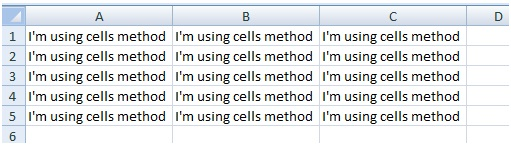Comments

You don’t need to use the Range object when you refer single cell using cells method. For e.g Thisworkbook.sheets(1).Cells(1,5). If you want to refer group of cells then you should use the Range method. Range method accept only two arguments for cells method[Range(Cells(1, 1), Cells(5, 3))]. First argument refer the top left of the range and second argument refer bottom right of the cell range.

### How to refer range using offset method?

Offset method is used to offset rows and column position from the targeted cell. Offset method accept two arguments. Offset(x,y)- where x represent the number of rows to offset or number of rows to move down(if x is positive) or move up( if x is negative) and y represent the number of columns to offset or number of columns to move right (if y is positive) or move left ( if y is negative)

Code

``````Sub Offset_method()

Range("A1").Offset(1, 2).Value = 1

Range("C1").Offset(1, -1).Value = 4

Range("D5").Offset(-2, -3).Value = 10

End Sub``````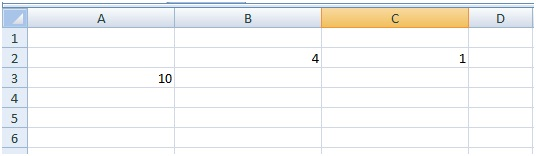### Recommended Excel VBA books

Excel 2016 Power Programming with VBA

Excel VBA 24-Hour Trainer

Excel VBA: A Beginners’ Guide